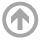Electrical and Computer Engineering Department

# EE353: Probability, Statistics and Linear Algebra for ECE

Course description: This course is an introduction to probability, statistics, and linear algebra with applications to electrical and computer engineering. The course begins with the fundamentals of probability theory and then relates these concepts to data analysis using the fundamentals of statistics. The course finishes with applications of linear algebra to solving engineering problems via matrix analysis and manipulation.

Course Objectives: By the end of the course, students shall be able to:

1. Use the fundamental properties and theorems of probability theory, sample spaces, events, and random variables to model real-life phenomena and to compute and interpret probabilities of events.
2. Apply statistical methods to estimate parameters of distributions from a representative sample of a population, and interpret the results.
3. Perform matrix computations and use eigenvalues and eigenvectors to analyze the structure of a matrix.

IEEE Code of Ethics